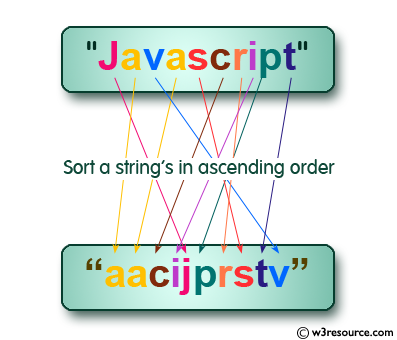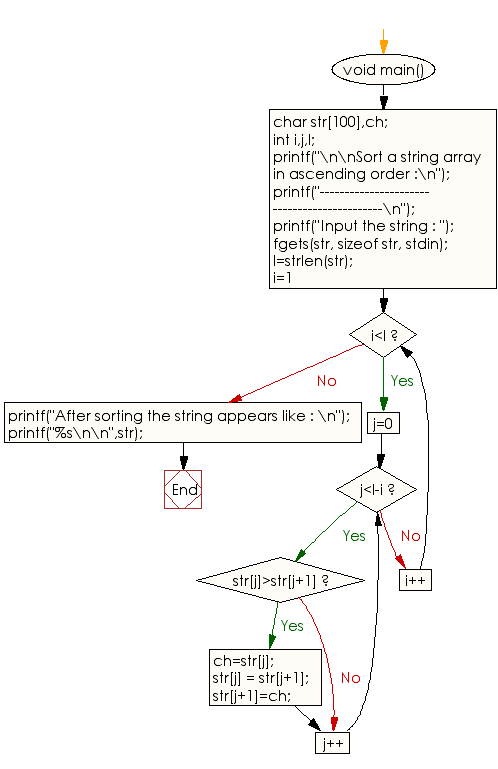﻿ C exercises: Sort a string array in ascending order - w3resource# C Programming: Sort a string array in ascending order

## C String: Exercise-11 with Solution

Write a C program to sort a string array in ascending order.Sample Solution:

C Code:

``````#include <stdio.h>
#include <string.h>
void main()
{
char str,ch;
int i,j,l;

printf("\n\nSort a string array in ascending order :\n");
printf("--------------------------------------------\n");
printf("Input the string : ");
fgets(str, sizeof str, stdin);
l=strlen(str);
/* sorting process */
for(i=1;i<l;i++)
for(j=0;j<l-i;j++)
if(str[j]>str[j+1])
{
ch=str[j];
str[j] = str[j+1];
str[j+1]=ch;
}
printf("After sorting the string appears like : \n");
printf("%s\n\n",str);
}
```
```

Sample Output:

```Sort a string array in ascending order :
--------------------------------------------
Input the string : w3resource
After sorting the string appears like :

3ceeorrsuw
```

Flowchart :C Programming Code Editor:

Improve this sample solution and post your code through Disqus.

What is the difficulty level of this exercise?

﻿